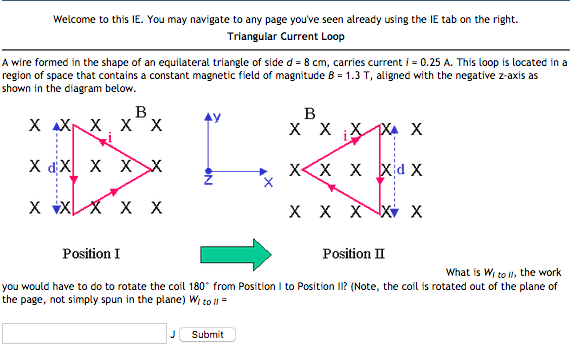# Question Welcome to this IE. You may navigate to any page you've seen already using the IE tab on the right. Triangular Current Loop A wire formed in the shape of an equilateral triangle of side d = 8 cm, carries current i = 0.25 A. This loop is located in a region of space that contains a constant magnetic field of magnitude B = 1.3 T, aligned with the negative z-axis as shown in the diagram below. B X X X X X X X X X X X X X X X ► x x x x x x x x x x xxx x x Position I Position II What is Wito I), the work you would have to do to rotate the coil 180° from Position to Position II? (Note, the coil is rotated out of the plane of the page, not simply spun in the plane) Wto 1 = J Submit